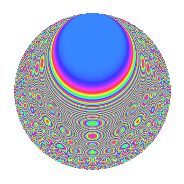# Properties

 Label 8044.2.aLevel 8044 Weight 2 Character orbit a Rep. character $$\chi_{8044}(1,\cdot)$$ Character field $$\Q$$ Dimension 167 Newform subspaces 2 Sturm bound 2012 Trace bound 1

# Related objects

## Defining parameters

 Level: $$N$$ = $$8044 = 2^{2} \cdot 2011$$ Weight: $$k$$ = $$2$$ Character orbit: $$[\chi]$$ = 8044.a (trivial) Character field: $$\Q$$ Newform subspaces: $$2$$ Sturm bound: $$2012$$ Trace bound: $$1$$

## Dimensions

The following table gives the dimensions of various subspaces of $$M_{2}(\Gamma_0(8044))$$.

Total New Old
Modular forms 1009 167 842
Cusp forms 1004 167 837
Eisenstein series 5 0 5

The following table gives the dimensions of the cuspidal new subspaces with specified eigenvalues for the Atkin-Lehner operators and the Fricke involution.

$$2$$$$2011$$FrickeDim.
$$-$$$$+$$$$-$$$$87$$
$$-$$$$-$$$$+$$$$80$$
Plus space$$+$$$$80$$
Minus space$$-$$$$87$$

## Trace form

 $$167q - 4q^{5} - 4q^{7} + 161q^{9} + O(q^{10})$$ $$167q - 4q^{5} - 4q^{7} + 161q^{9} + 2q^{11} - 2q^{13} - 8q^{15} - 4q^{17} + 4q^{19} - 6q^{21} - 4q^{23} + 151q^{25} + 6q^{27} - 10q^{29} - 10q^{31} + 2q^{33} - 4q^{35} - 22q^{37} - 20q^{39} + 2q^{41} - 6q^{43} - 28q^{45} - 16q^{47} + 143q^{49} + 18q^{51} + 6q^{53} - 18q^{55} - 20q^{57} + 6q^{59} - 26q^{61} - 22q^{63} - 14q^{65} + 10q^{67} - 20q^{69} + 14q^{71} + 2q^{73} + 12q^{75} + 8q^{77} - 36q^{79} + 143q^{81} - 16q^{83} - 4q^{85} + 2q^{87} - 4q^{89} + 26q^{91} + 36q^{95} + 2q^{97} + 4q^{99} + O(q^{100})$$

## Decomposition of $$S_{2}^{\mathrm{new}}(\Gamma_0(8044))$$ into newform subspaces

Label Dim. $$A$$ Field CM Traces A-L signs $q$-expansion
$$a_2$$ $$a_3$$ $$a_5$$ $$a_7$$ 2 2011
8044.2.a.a $$80$$ $$64.232$$ None $$0$$ $$-13$$ $$-2$$ $$-12$$ $$-$$ $$-$$
8044.2.a.b $$87$$ $$64.232$$ None $$0$$ $$13$$ $$-2$$ $$8$$ $$-$$ $$+$$

## Decomposition of $$S_{2}^{\mathrm{old}}(\Gamma_0(8044))$$ into lower level spaces

$$S_{2}^{\mathrm{old}}(\Gamma_0(8044)) \cong$$ $$S_{2}^{\mathrm{new}}(\Gamma_0(2011))$$$$^{\oplus 3}$$$$\oplus$$$$S_{2}^{\mathrm{new}}(\Gamma_0(4022))$$$$^{\oplus 2}$$